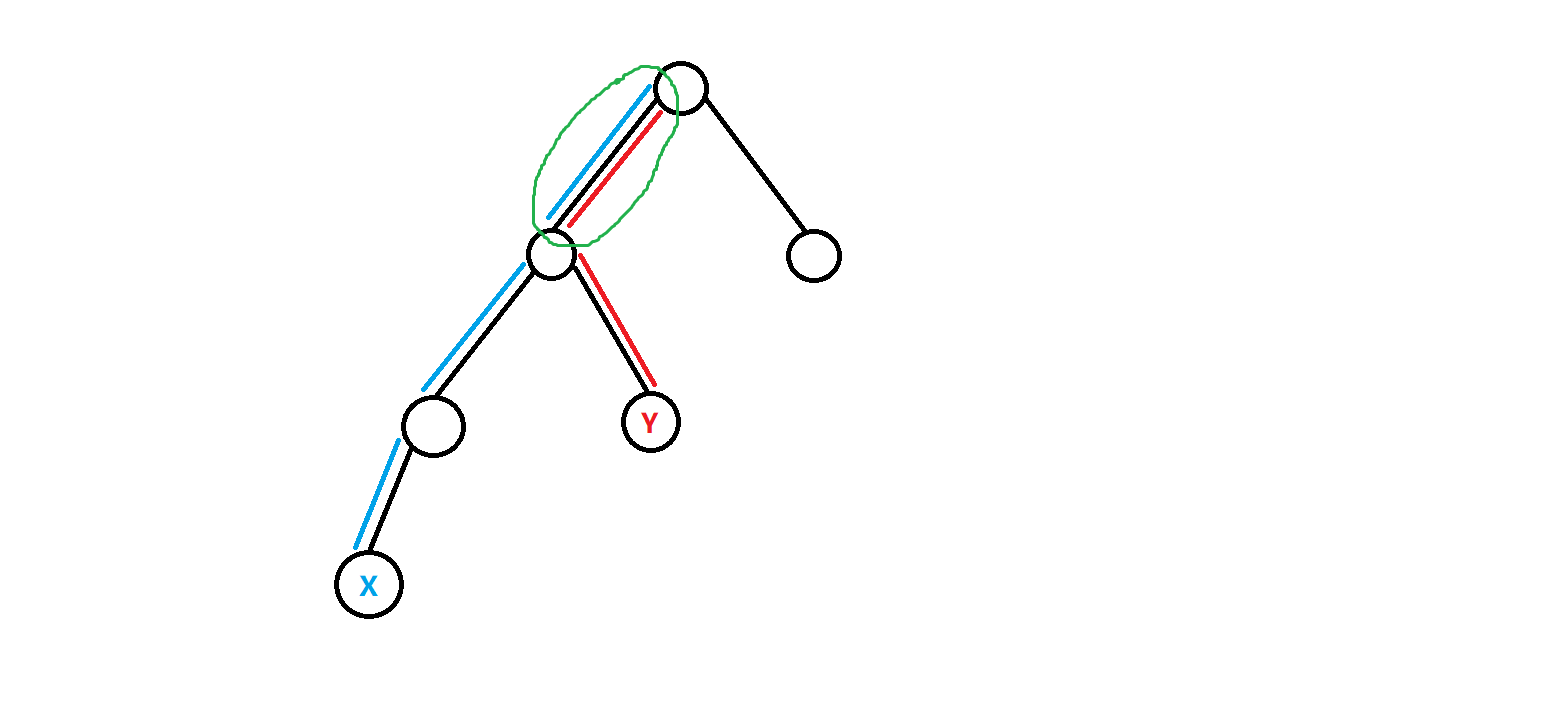# [點分治系列] 靜態點分

——正片開始——

O(nlogn)的優秀時間復雜度。

void getroot(int x,int fa){
siz[x]=1;son[x]=0;
int y=to(i);
if(y==fa||vis[y])continue;
getroot(y,x);
siz[x]+=siz[y];
if(siz[y]>son[x])son[x]=siz[y];
}
if(Siz-siz[x]>son[x])son[x]=Siz-siz[x];
if(son[x]<maxx)maxx=son[x],root=x;
}

update: 19.11.5 對于上面的代碼加一些解釋，Siz表示當前在求重心的這一棵樹的大小，root用來記錄重心

void getdis(int x,int fa,int d){//d表示x到目標點的距離
dis[++top]=d;//我們只需要知道每一個點到目標點的距離就行，不用知道這個點是哪個
int y=to(i);
if(y==fa||vis[y])continue;
getdis(y,x,d+val(i));
}
}void divide(int x){
vis[x]=1;//保證我們每次divide的x都是當前這棵樹的重心，所以標記x已經divide過了
solve(x,0,1);//計算這棵樹以x為重心的所有路徑答案
int totsiz=Siz;//這棵樹的大小
int y=to(i);
if(vis[y])continue;
solve(y,val(i),0);//求不合法路徑
maxx=inf,root=0;//初始化
Siz=siz[y]>siz[x]?totsiz-siz[x]:siz[y];//更新siz
getroot(y,x);//求出以y為根的子樹
divide(root);//以y為根的子樹分治下去
}
}

solve(y,val(i),-1);

void solve(int x,int d,int avl){//avl(available)表示這次計算得到的答案是否合法
top=0;//清空dis數組
getdis(x,0,d);//獲取到當前這棵樹到x的距離為d的所有dis
int cnt=0;
sort(dis+1,dis+top+1);//排好序準備二分
dis=-1;//第一個dis設置為奇怪的數方便下面比較
for(int i=1;i<=top;i++){//把所有距離相同的點放進一個桶里面方便操作
if(dis[i]==dis[i-1])
bucket[cnt].amount++;//原來桶的個數+1
else
bucket[++cnt].dis=dis[i],bucket[cnt].amount=1;//新開一個桶
}
for(int i=1;i<=m;i++){
if(query[i]%2==0)//如果k是偶數的話，我們單獨考慮一下距離為k/2那些點，它們可以互相配對形成長為k的路徑
for(int j=1;j<=cnt;j++)
if(bucket[j].dis==query[i]/2)//如果距離是k/2
ans[i]+=(bucket[j].amount-1)*bucket[j].amount/2*avl;
//組合計數，假設我們有x個距離為k/2的點，就有(x-1)*x/2個點對距離為k，也就是我們可以配出這么多個不同點對
//其實就是C(x,2)->x!/((x-2)!*2)->(x-1)*x/2
for(int j=1;j<=cnt&&bucket[j].dis<query[i]/2;j++){　　　　　　　 //接著枚舉<k/2的距離，然后我們二分找>2的距離配對，避免重復(點對(u,v)和(v,u)是等價的)，等于k/2的我們前面算過了，所以所有情況都考慮到了
int l=j+1,r=cnt;
while(l<=r){
int mid=(l++r)>>1;
if(bucket[j].dis+bucket[mid].dis==query[i]){
ans[i]+=bucket[j].amount*bucket[mid].amount*avl;
//組合計數記錄答案，假設我們有x個距離為m的點，y個距離為k-m的點，我們就有x*y個不同的點對(分類相乘)
break;//這一輪二分完了，下一輪
}
if(bucket[j].dis+bucket[mid].dis>query[i])r=mid-1;//大了，往小的二分
else l=mid+1;//小了，往大的二分
}
}
}
}

#include<bits/stdc++.h>
#define N 100010
#define lint long long
#define inf 0x7fffffff
using namespace std;
int vis[N],son[N],Siz,maxx,siz[N];
lint k;
struct Bucket{
int dis,amount;
}bucket[N];
struct Edge{
int nxt,to,val;
#define nxt(x) e[x].nxt
#define to(x) e[x].to
#define val(x) e[x].val
}e[N<<1];
int data=0,w=1;char ch=0;
while(ch!='-' && (ch<'0'||ch>'9'))ch=getchar();
if(ch=='-')w=-1,ch=getchar();
while(ch>='0' && ch<='9')data=data*10+ch-'0',ch=getchar();
return data*w;
}
inline void addedge(int f,int t,int val){
}
void getroot(int x,int fa){
siz[x]=1;son[x]=0;
int y=to(i);
if(y==fa||vis[y])continue;
getroot(y,x);
siz[x]+=siz[y];
if(siz[y]>son[x])son[x]=siz[y];
}
if(Siz-siz[x]>son[x])son[x]=Siz-siz[x];
if(son[x]<maxx)maxx=son[x],root=x;
}
void getdis(int x,int fa,int d){
dis[++top]=d;
int y=to(i);
if(y==fa||vis[y])continue;
getdis(y,x,d+val(i));
}
}
void solve(int rt,int d,int avl){//avl(available)表示這次計算得到的答案是否合法
top=0;//清空dis數組
getdis(rt,0,d);//獲取到當前這棵樹的rt的距離為d的所有dis
int cnt=0;
sort(dis+1,dis+top+1);//排好序準備二分
dis=-1;//第一個dis設置為奇怪的數方便下面比較
for(int i=1;i<=top;i++){//把所有距離相同的點放進一個桶里面方便操作
if(dis[i]==dis[i-1])
bucket[cnt].amount++;//原來桶的個數+1
else
bucket[++cnt].dis=dis[i],bucket[cnt].amount=1;//新開一個桶
}
for(int i=1;i<=m;i++){
if(query[i]%2==0)//如果k是偶數的話，我們單獨考慮一下距離為k/2那些點，它們可以互相配對形成長為k的路徑
for(int j=1;j<=cnt;j++)
if(bucket[j].dis==query[i]/2)//如果距離是k/2
ans[i]+=(bucket[j].amount-1)*bucket[j].amount/2*avl;
//組合計數，假設我們有x個距離為k/2的點，就有(x-1)*x/2個點對距離為k，也就是我們可以配出這么多個不同點對
//其實就是C(x,2)->x!/((x-2)!*2)->(x-1)*x/2
for(int j=1;j<=cnt&&bucket[j].dis<query[i]/2;j++){//接著枚舉<k/2的距離，然后我們二分找>2的距離配對
int l=j+1,r=cnt;
while(l<=r){
int mid=(l+r)>>1;
if(bucket[j].dis+bucket[mid].dis==query[i]){
ans[i]+=bucket[j].amount*bucket[mid].amount*avl;
//組合計數記錄答案，假設我們有x個距離為m的點，y個距離為k-m的點，我們就有x*y個不同的點對(分類相乘)
break;//這一輪二分完了，下一輪
}
if(bucket[j].dis+bucket[mid].dis>query[i])r=mid-1;//大了，往小的二分
else l=mid+1;//小了，往大的二分
}
}
}
}
void divide(int x){
vis[x]=1;
solve(x,0,1);//合法的算進去
int totsiz=Siz;
int y=to(i);
if(vis[y])continue;
solve(y,val(i),-1);//不合法的算出來減掉
maxx=inf,root=0;
Siz=siz[y]>siz[x]?totsiz-siz[x]:siz[y];
getroot(y,x);
divide(root);
}
}
int main(){
for(int i=1;i<n;i++){
}
maxx=inf;root=0;Siz=n;
getroot(1,0);
divide(root);
for(int i=1;i<=m;i++){
if(ans[i]>0)puts("AYE");
else puts("NAY");
}
return 0;
}

posted @ 2019-11-05 21:35  LightHouseOfficial  閱讀(...)  評論(...編輯  收藏
11选5走势图